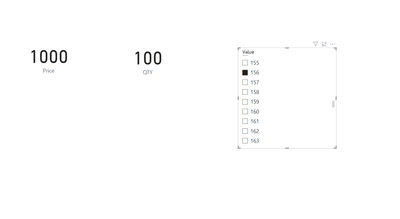cancel
Showing results for
Did you mean:

Fabric is Generally Available. Browse Fabric Presentations. Work towards your Fabric certification with the Cloud Skills Challenge.Frequent Visitor

## Finding nearest value from table with respect to selected value in slicer

Hi,
I have Created a Slicer for Entering a value by creating a calculated table :

Quantity Table = GENERATESERIES(0,90000,1)
I have a fact table - Rate Table
 Seq Qty Price 1 100 1000 2 250 1500 3 500 2000 4 1000 3000
Question is
How to find the nearest value of Qty and Price from the table with respect to the selected value in slicer ?

For eg : Entered Value in slicer = 1276
The Output : Qty 1000 and Price 3000
2 REPLIES 2Community Support

The approach @amitchandak  offers is fantastic,  In addition to referring to the solutions @amitchandak provides, you can also consider the following methods.

You can refer to the following measure

``````Price = VAR A=SELECTEDVALUE(Quantity[Value])
var c=SUMMARIZE(b,[Seq ],'Rate'[Qty],'Rate'[Price],[Divide],"Min",MINX(b,[Divide]))
return MINX(FILTER(c,[Min]=[Divide]),[Price])``````
``````QTY = VAR A=SELECTEDVALUE(Quantity[Value])
var c=SUMMARIZE(b,[Seq ],'Rate'[Qty],'Rate'[Price],[Divide],"Min",MINX(b,[Divide]))
return MINX(FILTER(c,[Min]=[Divide]),[Qty])``````

OutputBest Regards!

Yolo Zhu

If this post helps, then please consider Accept it as the solution to help the other members find it more quickly.Super User

@PowerBi_Xandar , create measures like

Qty M =
var _max = mInx(filter(Table, [Qty]>= selectedvalue('Quantity Table'[value])), Table[Qty])
var _min = Maxx(filter(Table, [Qty]<= selectedvalue('Quantity Table'[value])), Table[Qty])
return
calculate(Sum(Table[Qty]), filter(Table, Table[Qty] =if(_max -[Qty]> [Qty] -_min , _min, _max)))

Price M =
var _max = mInx(filter(Table, [Qty]>= selectedvalue('Quantity Table'[value])), Table[Qty])
var _min = Maxx(filter(Table, [Qty]<= selectedvalue('Quantity Table'[value])), Table[Qty])
return
calculate(Sum(Table[Price]), filter(Table, Table[Qty] =if(_max -[Qty]> [Qty] -_min , _min, _max)))

or use one of them a visual level filterAnnouncements#### Power BI Monthly Update - November 2023

Check out the November 2023 Power BI update to learn about new features.#### Fabric Community News unified experience

Read the latest Fabric Community announcements, including updates on Power BI, Synapse, Data Factory and Data Activator.#### The largest Power BI and Fabric virtual conference

130+ sessions, 130+ speakers, Product managers, MVPs, and experts. All about Power BI and Fabric. Attend online or watch the recordings.Top Solution Authors
Top Kudoed Authors
Users online (1,844)